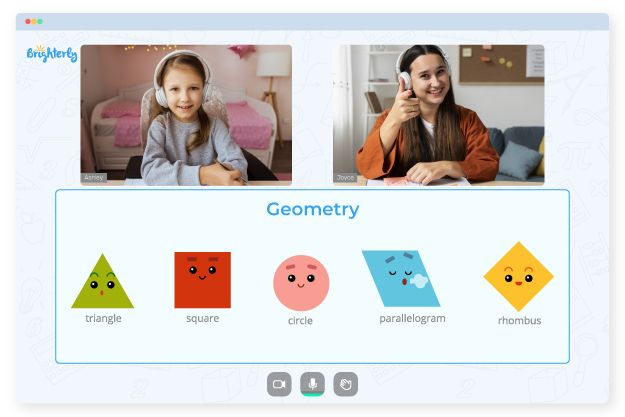In fourth grade, students should comprehend the concept of perimeter. It is the circumference of a shape that is frequently used to determine an object’s size or dimensions. But sometimes, understanding that concept doesn’t come easily to students. So, teachers frequently use 4th grade perimeter worksheets to teach and practice this idea with students.

## Getting the Best out of Perimeter Worksheets

Students in 4th grade practice and reinforce their understanding of perimeter using perimeter 4th grade worksheets. But to solve the questions in the worksheet, they must use their knowledge of perimeter from the previous grade.

Math for Kids

Is Your Child Struggling With Math?
1:1 Online Math TutoringFor teachers, there are several ways to get the best value out of the perimeter worksheet 4th grade. They can use the tools as part of class activity, tasking students with solving sections of the worksheet as a group. They can also use the worksheets to determine which students need more help or direction. Putting the students’ perimeter knowledge into practice using the 4th grade perimeter word problems worksheet is a great way for teachers to build their students’ confidence and proficiency in math.### Perimeter Worksheets Grade 4 PDF### Perimeter Worksheets Grade 4 PDF### Perimeter Worksheets Grade 4 PDF### Perimeter Worksheets Grade 4 PDF

4Th Grade Perimeter Word Problems Worksheet

Struggling with Geometry?• Does your child need extra support with understanding geometry concepts?
• Start lessons with an online tutor.

Is your child finding it hard to grasp the basics of geometry? An online tutor could be of help.